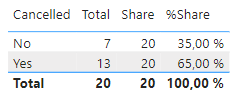cancel
Showing results for
Did you mean:Frequent Visitor

## Break down calculated share to date

Hi!

I have been using the basics of Power BI since a few months but I am relatively new to DAX. I have contract data and would like to calculate a cancellation rate for these.

These are an example how the data looks like:This is what I have created so far. I calclulated the Total sum first:

``Total = SUM('Tabelle1'[Count])``

Then I calculated the share and after that the percentage share:

``Share = CALCULATE([Total]; All('Tabelle1'[Cancelled]))``
``%Share = DIVIDE([Total]; [Share]; 0)``

My table in Power BI looks like this and that is working:But when I want to see the cancellation rate for the particular date, it does not work.I then had the idea to use ALLSELECT for this instead of ALL. Unfortunately, this didn't help either and I think I'm a bit lost and don't know what I'm doing.

I think it is a very simple question but I need some help 🙂

Markus

1 ACCEPTED SOLUTIONSuper User

@sukram1 , Try like

Divide(CALCULATE([Total]; Filter( 'Tabelle1','Tabelle1'[Cancelled] ="Yes") ),[Total])

or

Divide(CALCULATE([Total]; Filter( 'Tabelle1','Tabelle1'[Cancelled] ="Yes") ),calculate([Total],allselected()) )

!! Microsoft Fabric !!
Microsoft Power BI Learning Resources, 2023 !!
Learn Power BI - Full Course with Dec-2022, with Window, Index, Offset, 100+ Topics !!
Did I answer your question? Mark my post as a solution! Appreciate your Kudos !! Proud to be a Super User! !!
3 REPLIES 3Regular Visitor

Hey @sukram1
Please find below the base data, the measure and the two outputs (by Cancellation Status and by Date)
Please like If you find this helpful. The measure is quite simple. It will work automatically with the Yes/No status and DatesSuper User

@sukram1 , Try like

Divide(CALCULATE([Total]; Filter( 'Tabelle1','Tabelle1'[Cancelled] ="Yes") ),[Total])

or

Divide(CALCULATE([Total]; Filter( 'Tabelle1','Tabelle1'[Cancelled] ="Yes") ),calculate([Total],allselected()) )

!! Microsoft Fabric !!
Microsoft Power BI Learning Resources, 2023 !!
Learn Power BI - Full Course with Dec-2022, with Window, Index, Offset, 100+ Topics !!
Did I answer your question? Mark my post as a solution! Appreciate your Kudos !! Proud to be a Super User! !!Frequent Visitor

@amitchandak Thank you very much! This is exactly what I am looking for! It works perfect!!